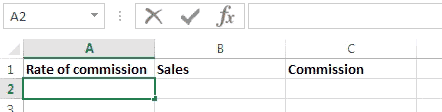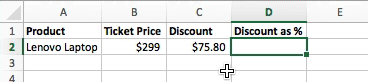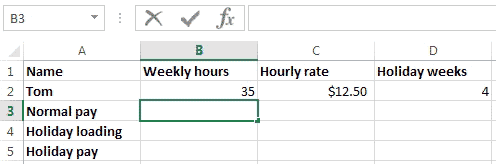### Excel formula

#### Commission | # gomaths.net/4221

Calculate the commission on sales.

`=A2*B2`

where `A2` is the rate of commission (entered as a percentage)
`B2` is the amount of sales#### Discount as percentage | # gomaths.net/4784

Calculate discount as a percentage from sale price and discount amount.

`=C2/B2`#### Holiday Pay | # gomaths.net/4220

Calculate the holiday pay.

`=0.175*B3`

where `B3` is 4 weeks normal pay